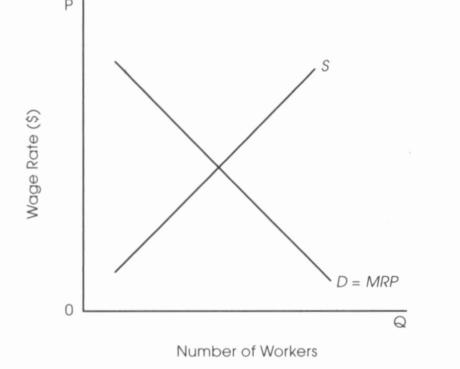# AP Microeconomics Practice Test 6

### Test Information12 questions14 minutes

1. Which of the following is NOT a characteristic of perfectly competitive industry?

2. Which of the following is a characteristic of monopolistic competition in the long run?

3. Which of the following is characteristic of oligopoly?

I. Formidable barriers to entry.

II. Price (P) = Marginal Revenue (MR).

III. Relatively few sellers.

4. Which of the following is a characteristic of monopoly?

5. Compared to perfect competition in the long run, monopoly has

6. With the presence of a negative externality, which of the following would internalize (or correct) the externality?

7. With the presence of a positive externality, which of the following would correct the externality?

8. Which of the following is true?

9. Which of the following is true?

10. Which of the following is true about the relationship of the average total cost (ATC) curve and the marginal cost (MC) curve?

11.Which of the following will happen when a new computerized system for a firm increases the marginal productivity of its workers?

12. Which of the following is true about a price floor?